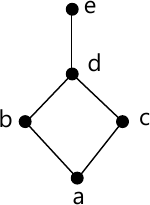MORE IN Discrete Mathematical Structures
VTU Computer Science (Semester 3)
Discrete Mathematical Structures
June 2014
Total marks: --
Total time: --
INSTRUCTIONS
(1) Assume appropriate data and state your reasons
(2) Marks are given to the right of every question
(3) Draw neat diagrams wherever necessary

1 (a) For any three set A,B,C, prove that A∩(B∪C)=(A∩B)∪(A∩C).
6 M
1 (b) Among the integers from 1 to 200, find the number of integers that are:
i) not divisible by 5
ii) divisible by 2 or 5 or 9
iii) not divisible by 2 or 5 or 9
7 M
1 (c) A problem is given to four students A,B,C,D whose chances of solving it are 1/2, 1/3, 1/4, 1/5 respectively. Find the probability that the problem is solved.
7 M

2 (a) Define a tautology and contradiction. Prove that, for any propositions p,q,r, the compound proposition [(p→q) ∧ (q→r)] → (p→r) is a tautology.
6 M
2 (b) Define the dual of logical statement. Verify the principle of duality for the following logical equivalence: [¬ (p∧q)→ ¬ p∨(¬ p∨q)]⇔ ( ¬ p∨q)
7 M
2 (c) Define converse, inverse and contra-positive of a conditional with truth table. Write down the contra-positive of [p→(q→r)] with:
i) only one occurrence of the connective →
ii) no occurrence of the connective →
7 M

3 (a) Negate and simplify each of the following:
i) ∃x, [p(x)∨q(x)]
ii) ∀x, [p(x)∧ ¬ q(x)]
iii) ∀x, [p(x)→q(x)]
6 M
3 (b) Establish the validity of the following argument:
[ egin {align*} &forall x, [p(x)vee q(x)] \ &forall x, [ left { eg p(x)wedge q(x) ight } o r(x)] \ hline& herefore forall x, [ eg r(x) o p(x)] end{align*} ]
6 M
3 (c) Prove that every even integer n with 2≤n≤26 can be written as a sum of atmost three perfect squares.
7 M

4 (a) Let a0=1, a1=2, a2=3 and an=an-1 +an-2+an-3 for n≥3. Prove that an=≤3n for all positive integrs n.
6 M
4 (b) Find an explicit definition of the sequence defined by a1=7, an=2an-1+1 for n≥2.
7 M
4 (c) The Lucas number are defined recursively by L0=2, L1=1 and Ln=Ln-1+L-2 for n≥2. Evaluate L2 to L10.
7 M

5 (a) Suppose A, B, C⊆Z X Y with A={(x,y)|y=5x-1}; B={(x,y)|y=6x}; C={(x,y)|3x-y=-7}. Find: [ i) Acap B \ ii) B cap C \ iii) overline{overline{A}cup overline{C}}\ iv) overline{B}cup overline{C} ]
6 M
5 (b) Define stirling number of second kind. Find the number of ways of distributing four distinct objects among three identical containers with some containers possibly empty.
7 M
5 (c) If f:A→B, g:B→C, and h:C→D are three functions then prove that (h⋅g)⋅f=h⋅(g⋅f)
7 M

6 (a) Let A={1,2,3,4}, B={w,x,y,z} and C={5,6,7}. Also let R1 be a relation from A to B defined by R1={(1,x), (2,x), (3,z)} and R2 and R3 be relation from B and C, defined by R2={(w,5),(x,6)}, R3={(w,5),(w,6)}. Find R1⋅R3.
6 M
6 (b) Find the number of equivalence relation that can be defined on a finite set A with |A|=6
7 M
6 (c) For A={a,b,c,d,e}, the Hasse diagam for the poset (A,R) is as shown below.
i) Determine the relation matrix for R.
ii) Construct the diagraph for R7 M

7 (a) Define subgroup of a group. Let G he a group and let J={x ∈ G|xy=yx for all y ∈ G}. Prove that J is a subgroup of G.
6 M
7 (b) State and prove Lagrange's theorem.
7 M
7 (c) The word C=1010110 is sent through a binary symmetric channel. If p=0.02 is the probability of incorrect receipt of a signal, find the probability that C is received as r=1011111. Determine the error pattern.
7 M

8 (a) The parity check matrix for an encoding function E: z23 → z26 given by [ H=egin{bmatrix} 1&0 &1 &1 &0 &0 \1 &1 &0 &0 &1 &0 \1 &0 &1 &0 &0 &1 end{bmatrix} ] i) Determine the associated generator matrix
ii) Does this code correct all single errors in transmission?
6 M
8 (b) Prove that the set z with binary operations ⊕ and ⊙ defined by x⊕y=x+y-1; x⊙y=x+y-xy is a cumulative ring.
7 M
8 (c) Show that z6 is no an integral domain.
7 M

More question papers from Discrete Mathematical Structures